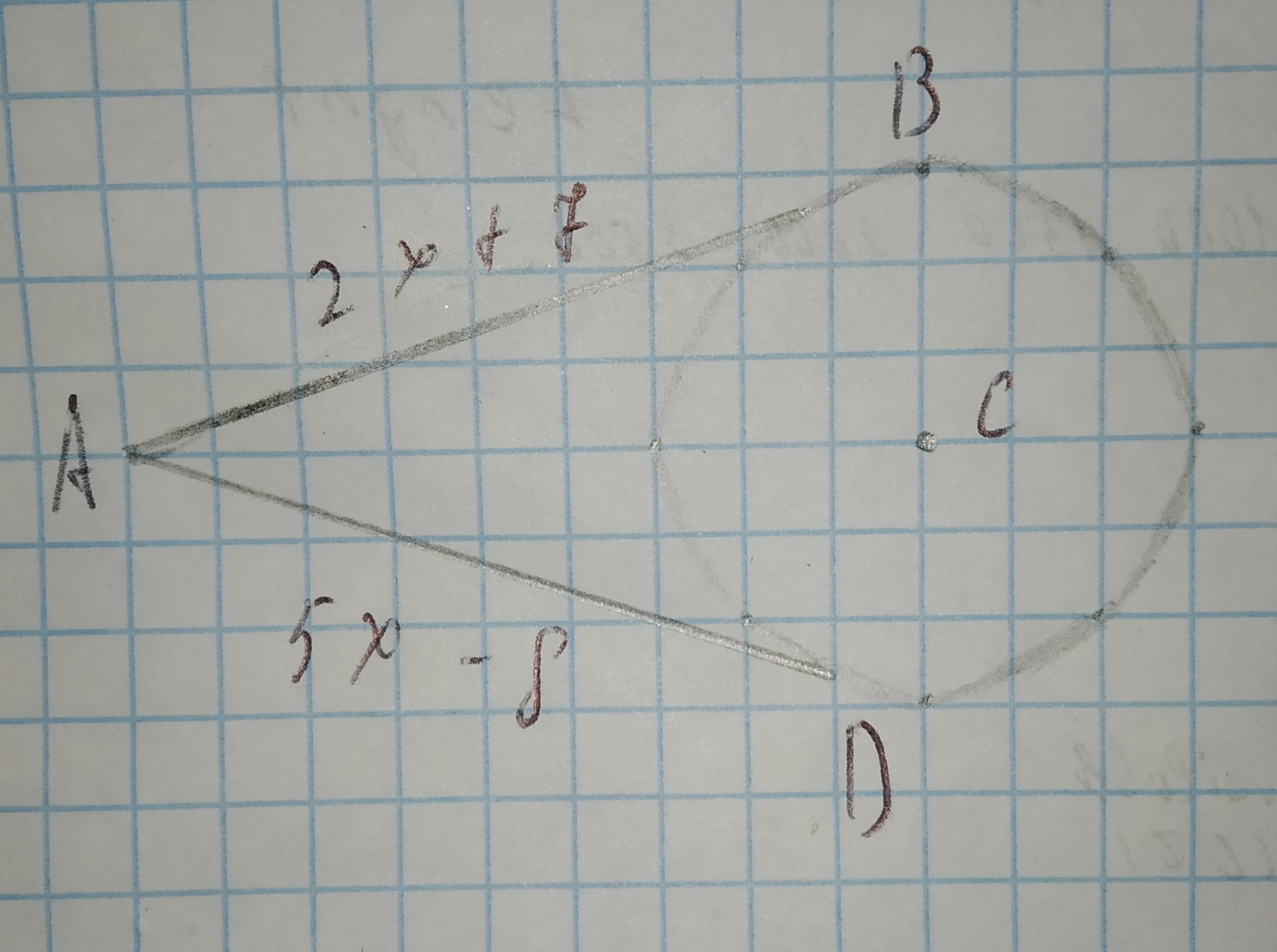Ask question

# Find the perimeter of the semicircular region. Round to the nearest hundredth.# Find the perimeter of the semicircular region. Round to the nearest hundredth.

Question
Circlesasked 2021-01-15
Find the perimeter of the semicircular region. Round to the nearest hundredth.

## Answers (1)2021-01-16
The perimeter of a semicircle region is the curved distance around the semicircle plus the diameter of the semicircle.
The curved distance around the semicircle is half of the circumference of the full circle. The formula for circumference is C=πd where d is the diameter. Half of the circumference would then be $$\displaystyle\frac{{1}}{{2}}π{d}$$. Since the diameter of the semicircle is 9 m, you need to substitute d=9 into $$\displaystyle\frac{{1}}{{2}}π{d}$$. This gives $$\displaystyle\frac{{1}}{{2}}π{\left({9}\right)}={4.5}π{m}.$$
The diameter of the semicircle is 9 m so the perimeter is then curved distance + diameter = $$\displaystyle{4.5}π+{94.5}π+{9}.$$ Using a calculator to evaluate gives $$\displaystyle{4.5}π+{9}≈{23.14}.$$ The perimeter of the semicircle is then about 23.14 m.

### Relevant Questionsasked 2021-05-16
Round 2093.33 to the nearest hundredth.asked 2020-11-08
The square surface cover shown in the figure is 10.16 centimeters on each side. The knockout in the center of the cover has a diameter of 1.27 centimeters. Find the area of the cover to the nearest hundredth centimeter.asked 2021-01-31
A museum groundskeeper is creating a semicircular statuary garden with a diameter of 25 feet. There will be a fence around the garden. The fencing costs $7.25$7.25 per linear foot. About how much will the fencing cost altogether? Round to the nearest hundredth. Use 3.14 for ππ. The fencing will cost aboutasked 2021-02-08
Find the distance UV between the points U(7,−4) and V(−3,−6). Round your answer to the nearest tenth, if necesasked 2020-10-20
Explain your reasoning, the distance around a circle a times the diameter the (5cm) Praciice and Problem Find the radius of the button.asked 2021-01-25
Point B is a point of tangency. Find the radius r of ☉C.asked 2021-02-06
Find the area of sector AOB if the radius of circle O is 12 cm. Give the answer in terms of π.asked 2020-12-28
Find the circumference of a circle with diameter 2/п.asked 2020-12-24
Points B and D are points of tangency. Find the value of x.asked 2021-02-18
A car’s rear windshield wiper rotates $$125^(circ)$$ The total length of the wiper mechanism is 25 inches and wipes the windshield over a distance of 14 inches. Find the area covered by the wiper.
...